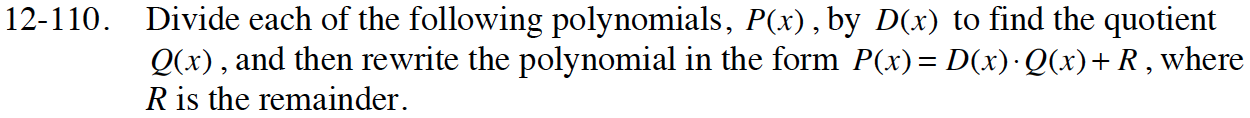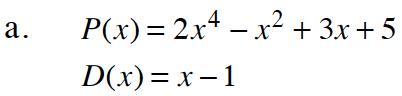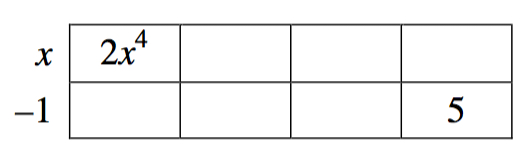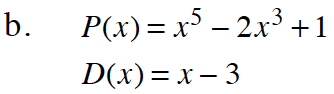Home > CCA2 > Chapter 12 > Lesson 12.2.2 > Problem12-110

12-110.
1. Divide each of the following polynomials, P(x), by D(x) to find the quotient Q(x) and then rewrite the polynomial in the form P(x) = D(x) · Q(x) + R, where R is the remainder. Homework Help ✎

1. P(x) = 2x4x2 + 3x + 5
D(x) = x − 1

2. P(x) = x5 − 2x3 + 1
D(x) = x − 3P(x) = (x − 1)(2x3 + 2x2 + x + 4) + 9P(x) = x5 + 0x4 − 2x3 + 0x2 + 0x + 1